Function Repository Resource:

# ComplexBubblePlot

Visualize a complex function as an array of bubbles

Contributed by: Jan Mangaldan
 ResourceFunction["ComplexBubblePlot"][f,{z,zmin,zmax}] generates a plot of f as an array of disks scaled by Abs[f], over the complex rectangle with corners zmin and zmax.

## Details and Options

In a complex bubble plot, each disk has its center at {Re[f[z]],Im[f[z]]}, with the radius a scaling of Abs[f[z]], and colored by Arg[f[z]].
ResourceFunction["ComplexBubblePlot"][f,{z,n}] is equivalent to ResourceFunction["ComplexBubblePlot"][f,{z,-n-n I,n+n I}].
ResourceFunction["ComplexBubblePlot"] treats the variable z as local, effectively using Block.
ResourceFunction["ComplexBubblePlot"] has attribute HoldAll and evaluates f only after assigning specific numerical values to z. In some cases, it may be more efficient to use Evaluate to evaluate f symbolically first.
ResourceFunction["ComplexBubblePlot"] has the same options as Graphics, with the following additions and changes:
 ColorFunction Automatic how to apply coloring to disks ColorFunctionScaling True whether to scale arguments to ColorFunction Frame Automatic whether to put a frame around the plot PlotLegends None legends for color gradients PlotPoints Automatic the number of disks in each direction PlotRange Automatic range of values to include PlotRangeClipping True whether to clip at the plot range WorkingPrecision MachinePrecision the precision used in internal computations
ColorFunction is supplied with a single argument, given by default by the scaled value of Arg[f[z]].

## Examples

### Basic Examples (2)

Plot a complex function:

 In:=Out=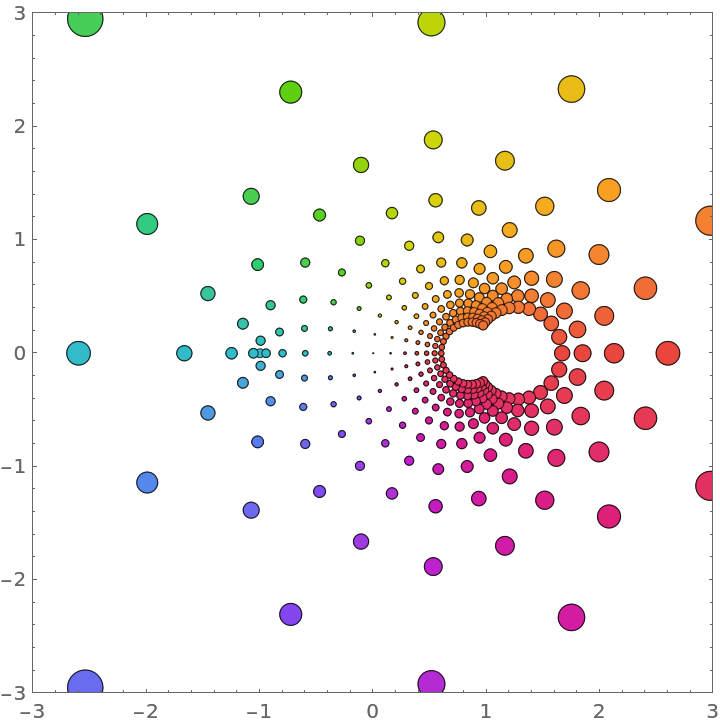Include a legend showing how the colors vary from -π to π:

 In:=Out=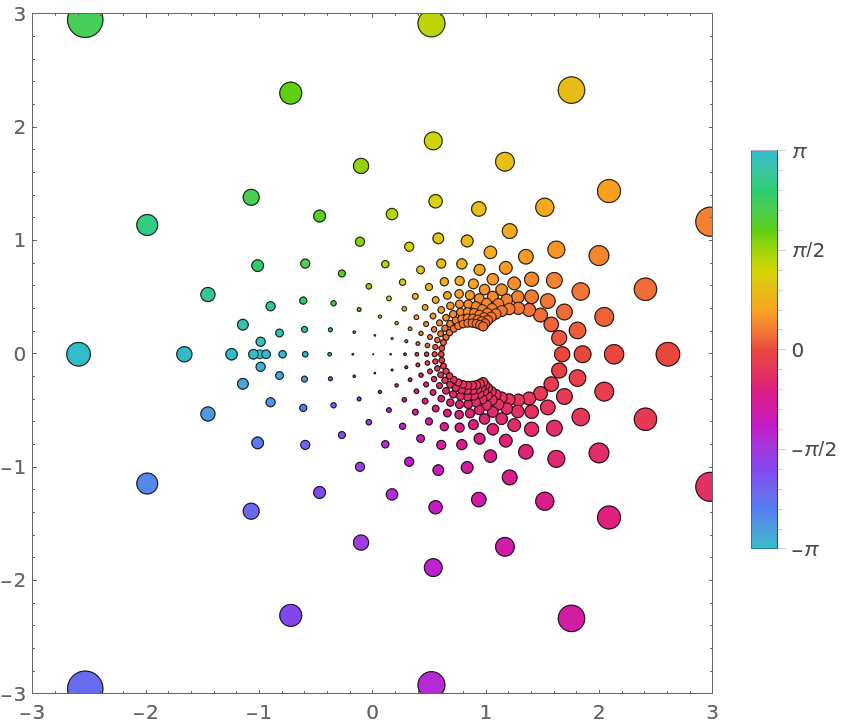### Scope (3)

The identity function:

 In:=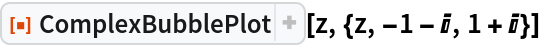Out=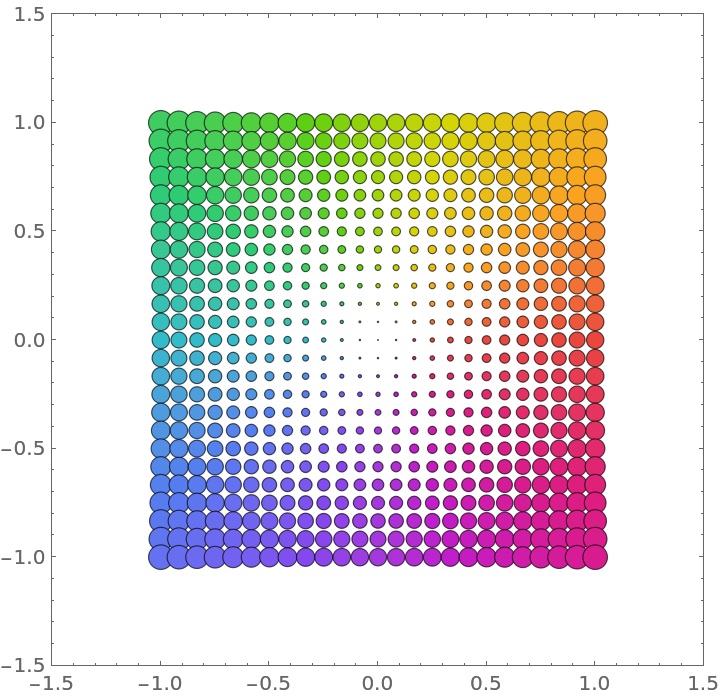Visualize various Power functions:

 In:=Out=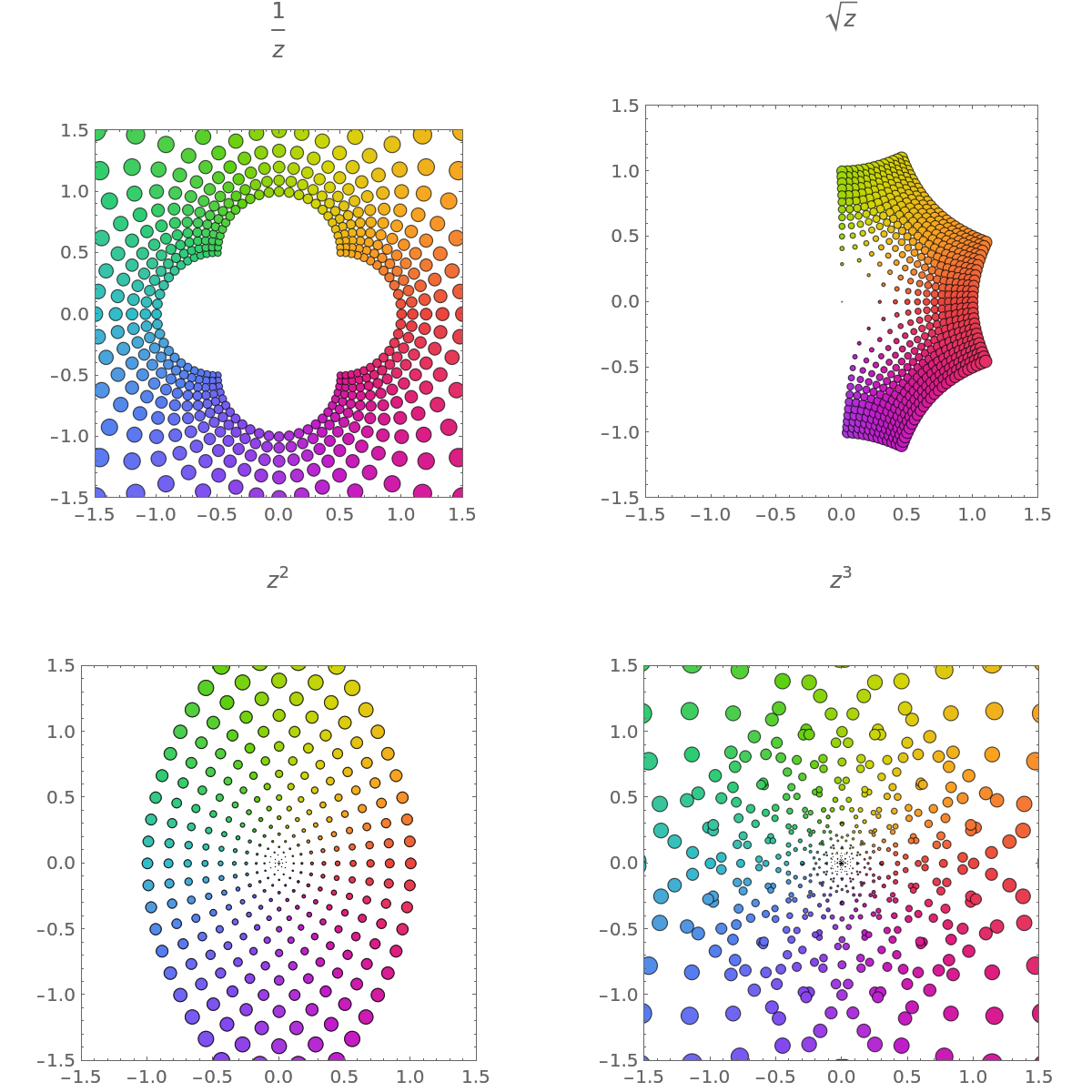Visualize a function with an essential singularity:

 In:=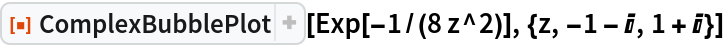Out=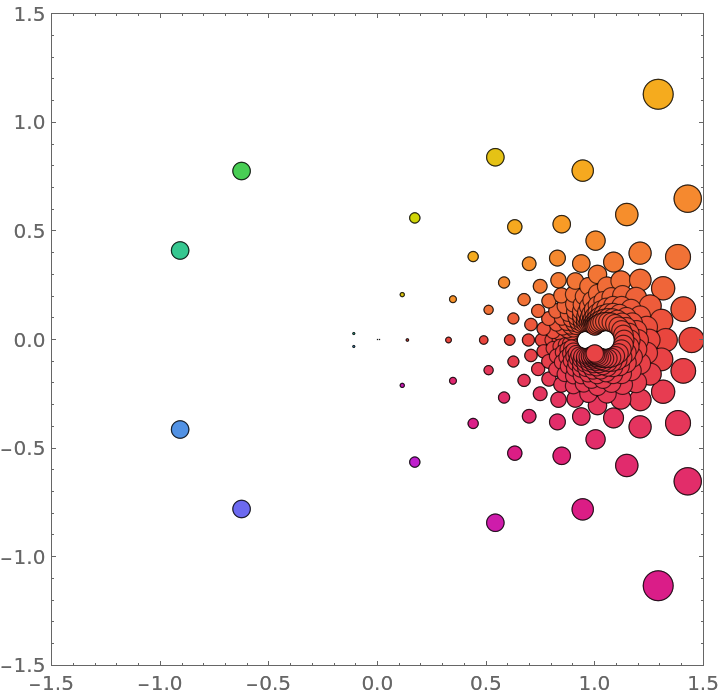### Options (5)

#### ColorFunction (1)

Use a different color function:

 In:=Out=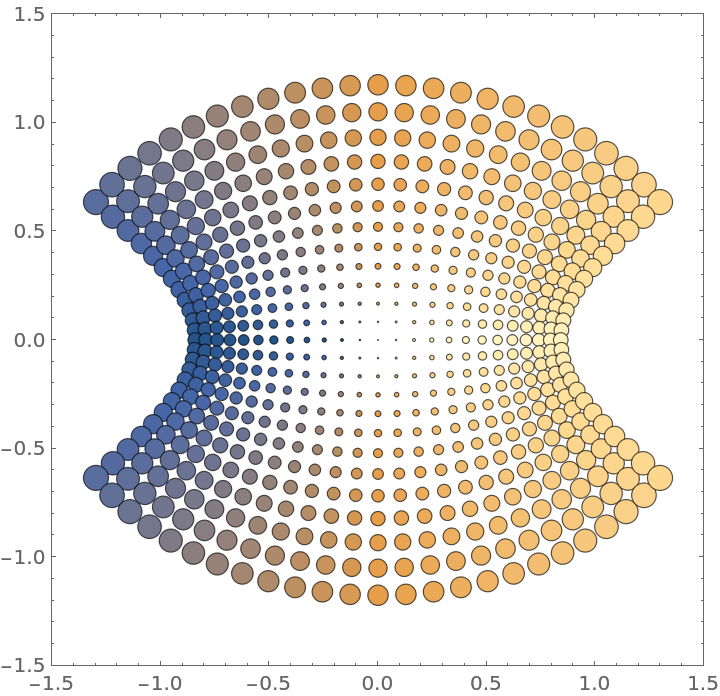#### ColorFunctionScaling (1)

Arg[f] is scaled by default. Use ColorFunctionScaling to change it:

 In:=Out=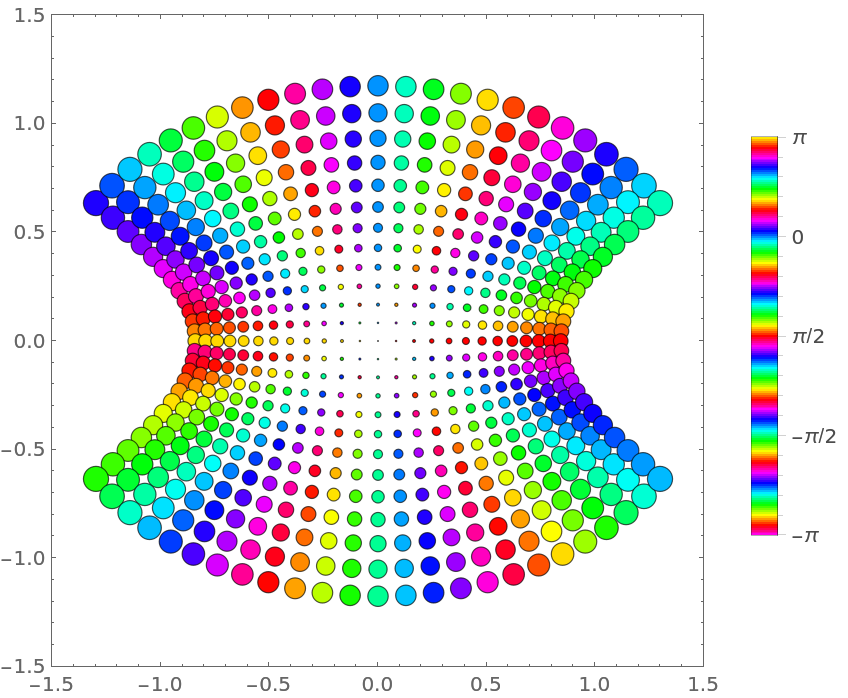#### PlotLegends (1)

The Automatic legend shows the association between color and phase:

 In:=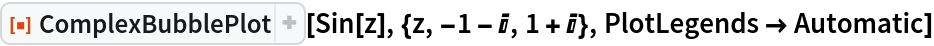Out=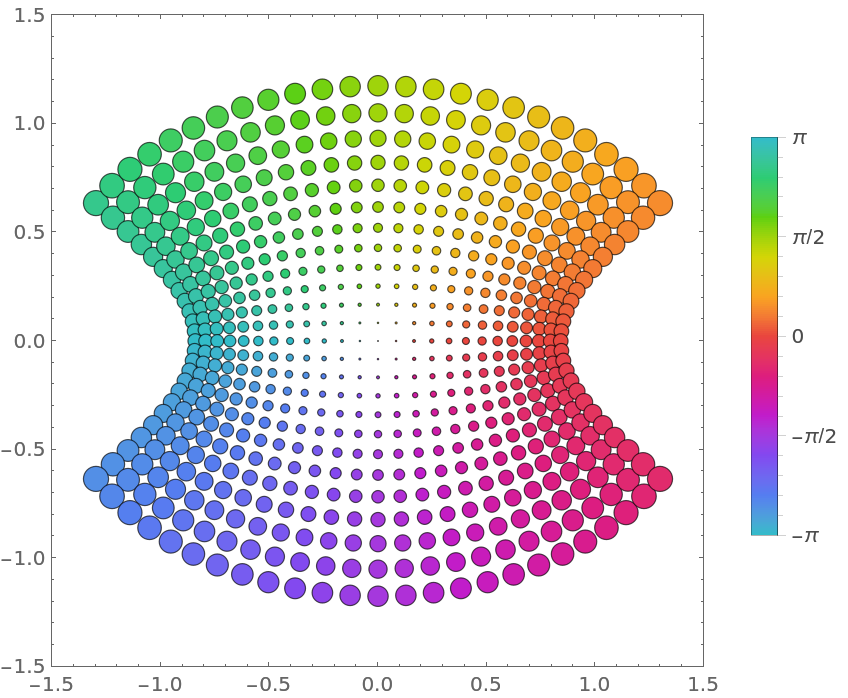#### PlotPoints (1)

Use more disks:

 In:=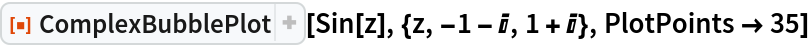Out=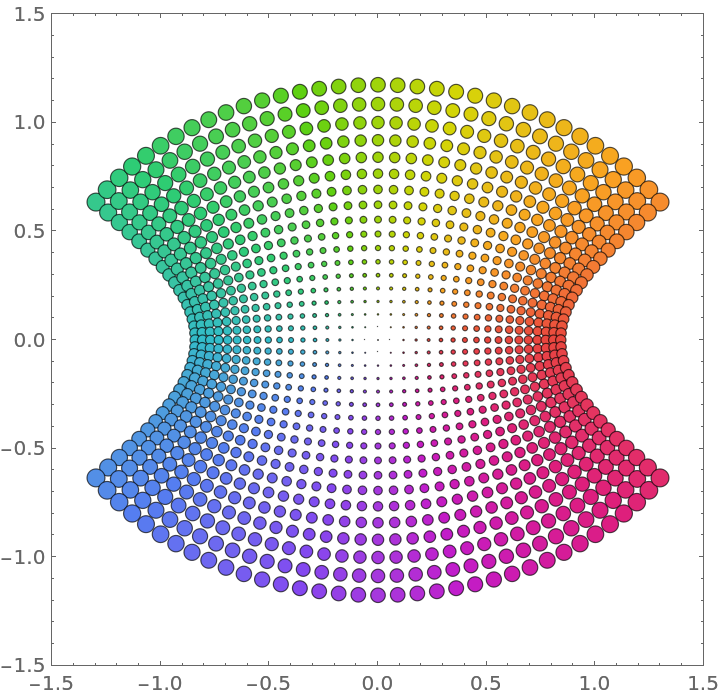#### WorkingPrecision (1)

Evaluate functions using arbitrary-precision arithmetic:

 In:=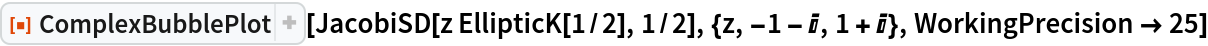Out=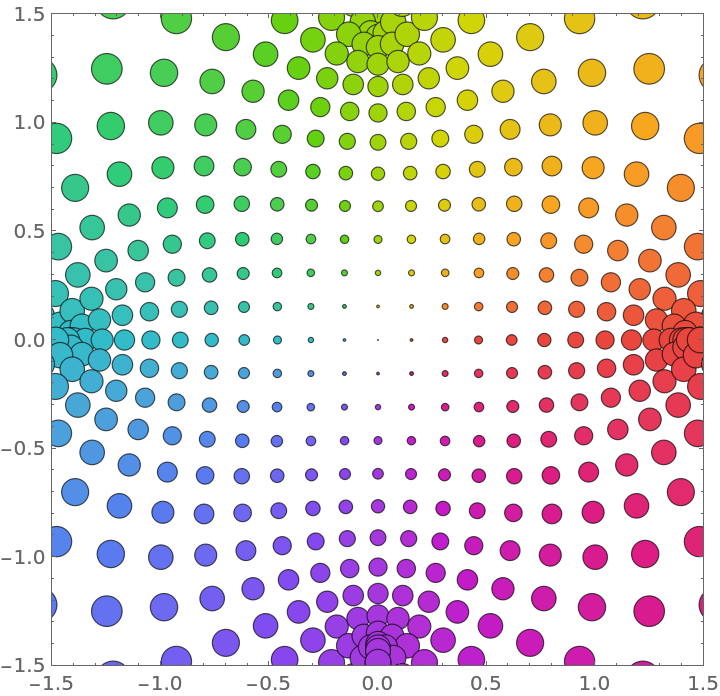### Neat Examples (1)

Visualize the function -ⅈza with varying a:

 In:=Out=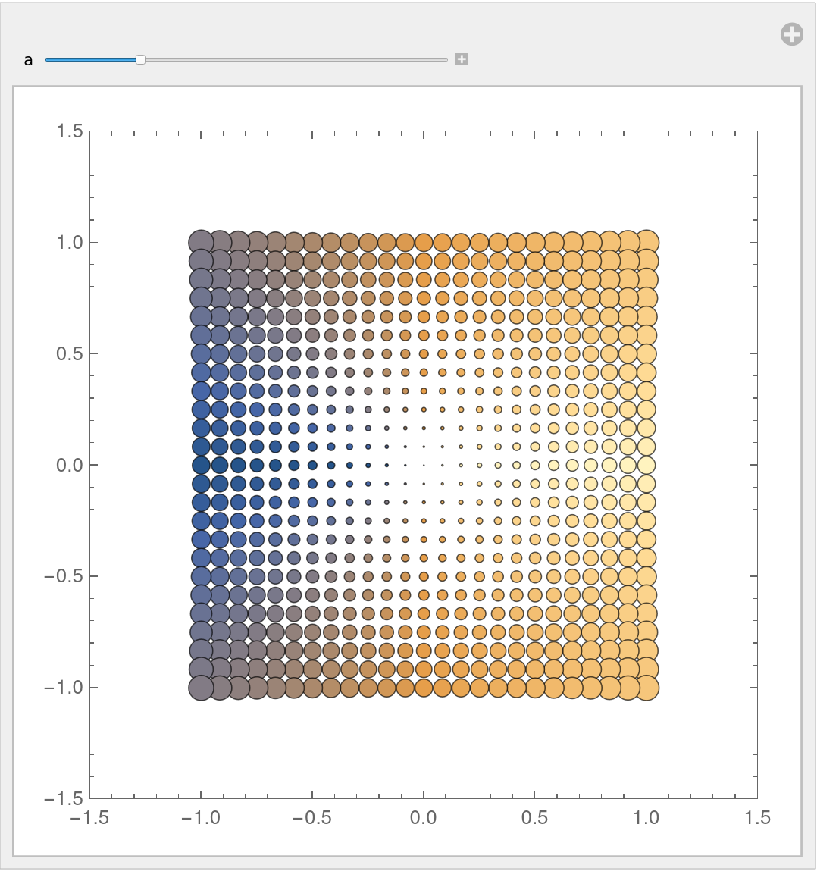## Version History

• 1.0.0 – 20 January 2021